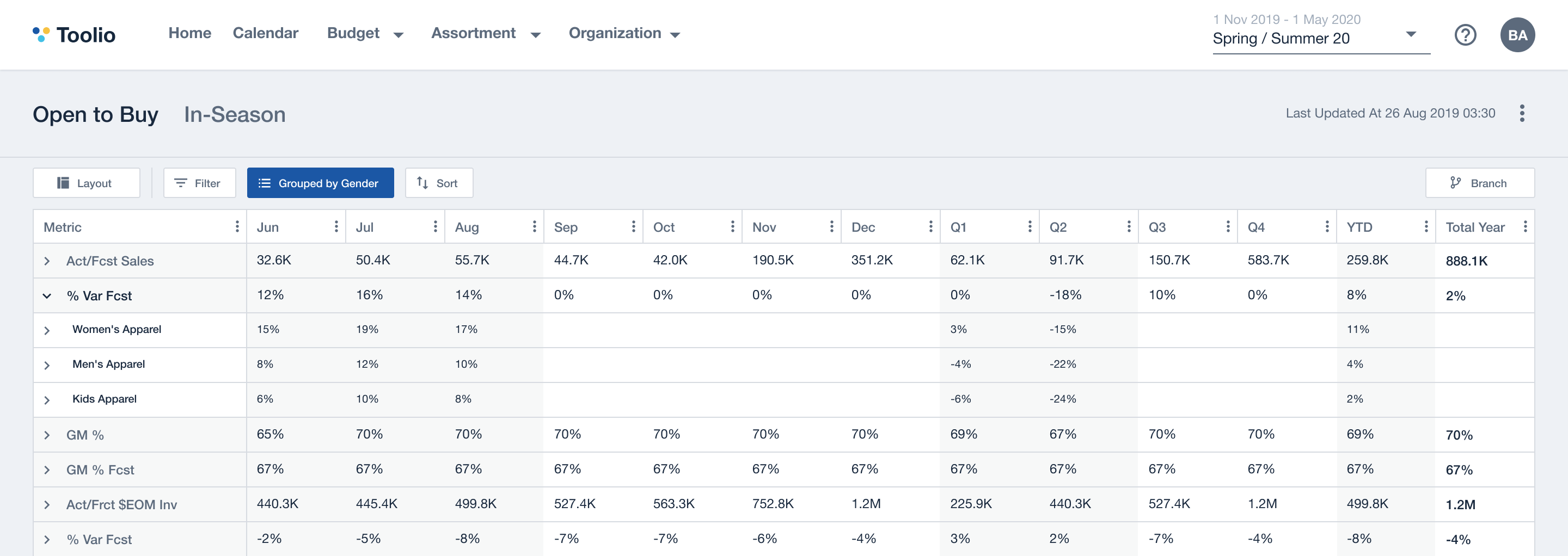CEO & Co-Founder

# Retail Math FundamentalsBelow are the retail formulas we look at closely when we are evaluating the performance of a retailer's sales and inventory utilization.

Average dollar transaction during a period of time

ADS = Net Sales \$ / Number of Transactions

#### Average Unit Retail (AUR)

Average selling price of an item

Sales AUR = Net Sales \$ / Units Sold
Inventory AUR = Retail Inventory / Units OH

#### Average Unit Cost (AUC)

Average cost per unit

Sales AUC  = COGS / Units Sold
Inventory AUC = Inventory at Cost / Units OH

#### Unit per Transaction (UPT)

Average units sold per transaction

UPT = Units Sales / Number of Transactions

#### Stock to Sales (S/S)

Number of weeks of inventory on hand. Based on prior weeks sales. Can be calculated at a monthly level

S/S = Inventory Beginning of Period / Prior Week Sales

#### Sell Through (ST)

The percent of inventory sold in a given period time. Mostly commonly a week.

ST = Units Sold / BOP On Hand In-Store Units

#### Weeks of Supply (WOS)

Number of weeks a given amount of inventory will last

WOS = Currently Inventory Units / Average Weekly Unit Sales

#### Build

Relationship of current time period sales to the prior time sales period (expressed as a percentage)

Build = Total Sales Retail / Previous Total Sales Retail - 1

#### Comparable Store Sales (Comps)

Comparison of sales for stores that have been open more than a year.

#### Average Inventory

Calculated in dollars or units for any period of time

Average Inventory = (Sum or all BOP inventory + EOP Inventory of the last period) / The Number of Data Points Used

#### Turn

Number of time the average inventory is sold and replaced during a specific time period.

Turn = Net Sales (Cost\$ or Units) / Average Inventory (Cost\$ or Units)

#### Initial Markup (IMU)

Difference between the unit cost and the ticket price (expressed as a %)

IMU% = (Ticket\$ - Cost\$) / Ticket\$ * 100%

#### Maintained Mark-Up (MMU)

The profit of an item after all markdowns, allowances and cancellations have deducted

MMU\$ = IMU Retail \$ - Markdowns at Cost - Other Costs
MMU% = Maintained Margin Dollars / Net Sales Retail

#### Gross Margin Return on Investment (GMROI)

Gross margin dollars returned for every dollar invested in inventory. This is used as a measure of productivity of a particular category or SKU, i.e. investment area and makes a great benchmark between product groups or even between companies.

GMROI = Gross Margin \$ / Average Inventory at Cost